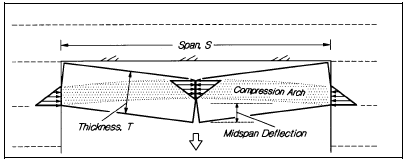The Rocscience International Conference 2021 Proceedings are now available. Read Now

# Arch Snap-Thru Probability

This is the primary failure mode of interest in a Voussoir analysis. The essential feature of a roof supported by a Voussoir action are illustrated in the figure below, for a horizontal beam. Deflection has been exaggerated for purposes of illustration.

Problem geometry for Voussoir stability analysis (Hutchinson et al., 1996)Like elastic buckling, "arch snap-thru" is highly dependent on geometry (span/depth ratio) and rock mass modulus. However, the driving force for gravity buckling is the self-weight of the rock, whereas the driving force for elastic buckling is the externally applied lateral stress. As such, they are entirely different modes of failure, although both are referred to as buckling failure modes.

The buckling parameter calculated in a Voussoir analysis represents the percentage of unstable arch configurations for a given geometry and rock mass modulus, as determined by the Voussoir analysis iteration procedure. A buckling parameter = 35% has been determined (Hutchinson et al., 1996) as a limit above which a roof should be considered unstable. This design limit of 35% happens to correspond to midspan deflection = 10% of the beam thickness. Therefore arch stability can also be assessed by monitoring the displacement at midspan, relative to the undeflected state. The AVERAGE displacement at midspan (for all statistical combinations of input parameters) is displayed along with the arch snap-thru statistics.

"Failure Probability" display

The display of "failure probability" is slightly different than that used for other CPillar failure modes. The shaded portion of the distribution curve indicates buckling parameters > 35 (rather than factor of safety values < 1). Also, the failure probability is stated as either: LOW, MEDIUM, HIGH, or VERY HIGH, rather than a precise numerical value, due to the nature of a Voussoir stability analysis. These failure probabilities correspond to mean buckling parameter ranges as indicated in the table below.

Failure probabilities (Voussoir analysis) corresponding to mean buckling parameter ranges

 Failure Probability Mean Buckling Parameter LOW 0-10 MEDIUM 10-25 HIGH 25-50 VERY HIGH > 50

Note

The design curves of Beer & Meek (1982) correspond to the CPillar solution when the buckling parameter (in CPillar) just reaches 100 (i.e.. 99 to 100). This represents a mathematically critical state which will lead to collapse at the slightest disturbance, and as such is not a conservative design limit. The CPillar recommended limit for arch snap-thru stability (buckling parameter < 35) is based on a more conservative but realistic approach to the voussoir concept of roof stability. See Hutchinson et al. (1996) for full details.